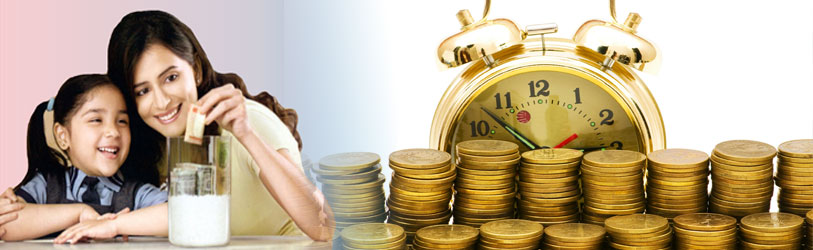Our SchemesFixed Deposite Scheme

Maximum Interest Rate (On 5 Year Deposit) 12 .50 %

Fixed Deposit Scheme (FD)

 Time Interest Rate Maturity Amount 6 Month 8.25% Rs. 10,416.75 1 Year 11.25% Rs. 11,173.36 2 Year 11.50% Rs. 12,545.23 3 Year 11.75% Rs.14,154.14 4 Year 12.00% Rs.16,047.06 5 Year 12.50% Rs.18,504.58Monthly Income Scheme

Deposit Rs. 10,750 and get 20,018 after 60 months.

Monthly Income Scheme (MIS)

 Time Interest Rate Maturity Amount 1 Year 11.25% Rs. 93.75 2 Year 11.25% Rs.93.75 3 Year 11.75% Rs. 97.92 4 Year 11.75% Rs. 97.92 5 Year 12.50% Rs.103.09Recurring Deposit Scheme

Recurring Deposit Scheme (RD) 5 Year

 Monthly Deposit Scheme Total Deposit scheme Interest Amount Maturity Amount Rs. 100 Rs. 6000 Rs. 1636 Rs. 7636 Rs. 200 Rs. 12000 Rs. 3272 Rs.15,272 Rs. 500 Rs. 30000 Rs.8181 Rs.38,181 Rs. 1000 Rs. 60000 Rs.16363 Rs.76,363 Rs. 1500 Rs. 90000 Rs.24545 Rs.1,14,545

Recurring Deposit Scheme (RD) 3 Year

 Monthly Deposit Scheme Total Deposit scheme Interest Amount Maturity Amount Rs.300 Rs. 10,800 Rs. 1431 Rs.12,231 Rs.500 Rs.18,000 Rs.2386 Rs.20,836 Rs.1000 Rs.36000 Rs.4772 Rs.40,772 Rs.1500 Rs.54000 Rs.7159 Rs.61,159

Recurring Deposit Scheme (RD) 2 Year

 Monthly Deposit Scheme Total Deposit scheme Interest Amount Maturity Amount Rs.500 Rs.12000 Rs.910 Rs.12,910 Rs.1000 Rs,24000 Rs.1820 Rs.25,820 Rs.1500 Rs.36000 Rs.2730 Rs.38,730

Recurring Deposit Scheme (RD) 1 Year

 Monthly Deposit Scheme Total Deposit scheme Interest Amount Maturity Amount Rs.1000 Rs. 12000 Rs.262 Rs.12,262 Rs.1500 Rs.18000 Rs.393 Rs18,393 Rs.5000 Rs.60000 Rs.1312 Rs.61,312Daily Deposit Scheme

Daily Deposit Scheme (DDS) 1 Year (365 Days)

Daily Deposit Scheme (DDS)

 Monthly Deposit Scheme Total Deposit scheme Interest Amount Maturity Amount Rs.50 Rs.18250 Rs.350 Rs.18,600 Rs.100 Rs.36500 Rs.700 Rs.37,200 Rs.200 Rs.73000 Rs.1400 Rs.74,400

Daily Deposit Scheme (DDS) 2 Year (365 Days)

 Monthly Deposit Scheme Total Deposit scheme Interest Amount Maturity Amount Rs.50 Rs.36500 Rs.800 Rs.37,300 Rs.100 Rs.73000 Rs.1600 74,600 Rs.200 Rs.1,46000 Rs.3200 Rs.1,49,200Saving Account

Minimum balance is 1000/-
Rate of interest 6%.